##### Microeconomics For Dummies - UK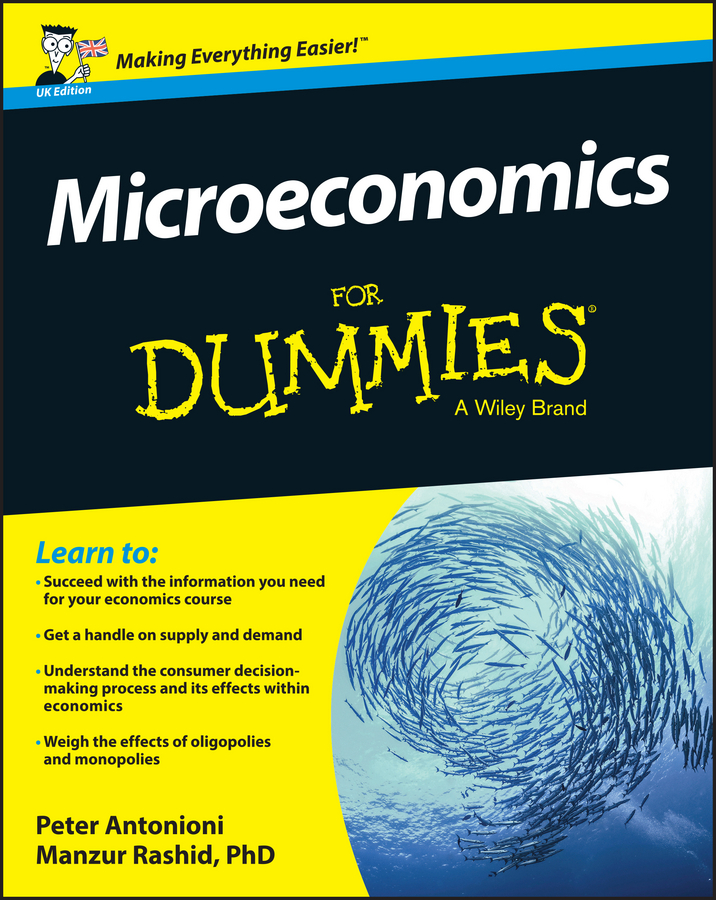The Cournot and Stackelberg duopoly theories in managerial economics focus on firms competing through the quantity of output they produce. The Bertrand duopoly model examines price competition among firms that produce differentiated but highly substitutable products. Each firm’s quantity demanded is a function of not only the price it charges but also the price charged by its rival. Coca-Cola and Pepsi are examples of Bertrand duopolists.

With the Bertrand model, you focus on what price is selected to maximize your profits. In the Cournot and Stackelberg duopoly models, the focus is on quantity.

The quantity demanded for firm A and firm B is a function of both the price the firm establishes and the price established by their rival because the goods are highly substitutable. Thus, the firms have the following demand curves relating quantity demanded to its price and its rival’s price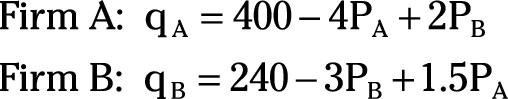To simplify the analysis, assume that both firms have zero marginal cost for their products. Profit maximization then requires each firm to choose a price that maximizes its total revenue.

Derive the Bertrand reaction functions for each firm with the following steps:

1. Firm A’s total revenue equals price times quantity, so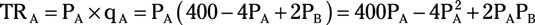2. Taking the derivative of firm A’s total revenue with respect to the price it charges yields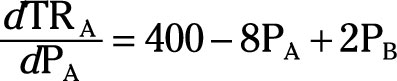3. Setting the equation in Step 2 equal to zero and solving it for PA generates firm A’s reaction function.

Setting the derivative of total revenue equal to zero maximizes total revenue, which also maximizes profit given marginal cost equals zero.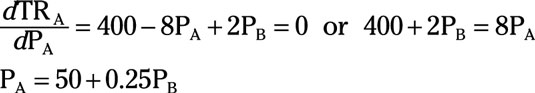4. Repeat these steps for firm B to derive its reaction function.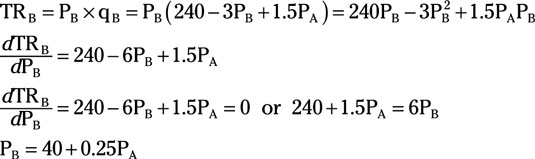5. Substitute firm B’s reaction function into firm A’s reaction to determine PA.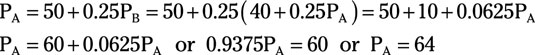6. Substitute PA equals 64 in firm B’s reaction function to determine PB.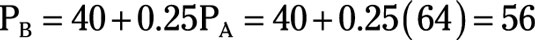The Bertrand duopoly model indicates that firm A maximizes profit by charging \$64, and firm B maximizes profit by charging \$56. Note that both the horizontal and vertical axes on the illustration measure price and not quantity (as in the Cournot and Stackelberg models).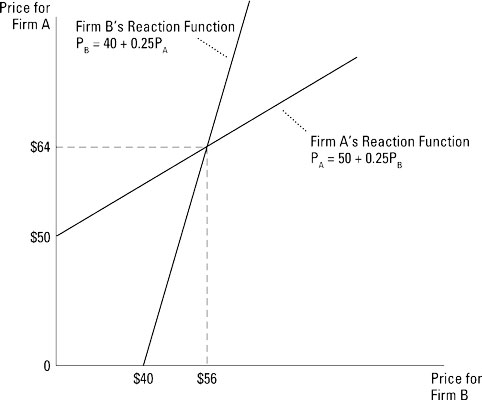In the Bertrand model, firms compete with price. Therefore, reaction functions are expressed in terms of price, not quantities.